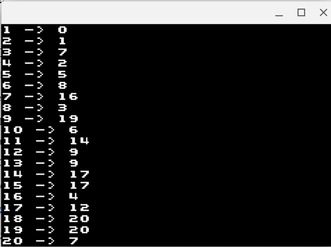# Collatz Exercise

## Objective

To get experience writing ARM assembly code for the GBA.

## The Collatz Conjecture

The Collatz conjecture states that if you take any positive integer $N$ and repeatedly follow the following process:
1. If $N$ is even, divide it by 2.
2. Otherwise multiply it by 3 and add 1.

Eventually $N$ will reach 1.

For instance, if we start with $N = 10$, then we get the following sequence of numbers:

10
5
16
8
4
2
1


The number of steps it takes for 10 to reach 1 is 6.

This conjecture has been shown to hold true for numbers up to $2^{60}$ but has never been proven.

For this lab, you will write an ARM assembly function to calculate the number of steps it takes any number to reach 1.

wget cs.umw.edu/~finlayson/class/spring18/cpsc305/labs/collatz.tar.gz
tar xvf collatz.tar.gz

2. Compile the program so that you can see you have everything set up correctly:
gbacc collatz.s main.c

3. Run the program.gba program this creates. It should print a list of 20 results "1 -> 1", "2 -> 2" etc. The empty collatz function just returns its argument unchanged.
4. Edit the collatz function in collatz.s to do the calculation as described above.

When you are done, your program should produce the following output:## Submitting

When your program works, email the .s file to ifinlay@umw.edu.